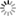##### Personal tools
You are here: Home Matrix_Linear_Algebra_111720A

# Matrix_Linear_Algebra_111720A

[An m × n matrix: the m rows are horizontal and the n columns are vertical. Each element of a matrix is often denoted by a variable with two subscripts. For example, a2,1 represents the element at the second row and first column of the matrix.]
##### Document Actions